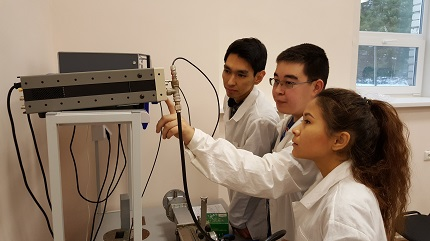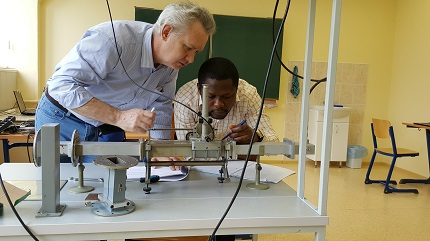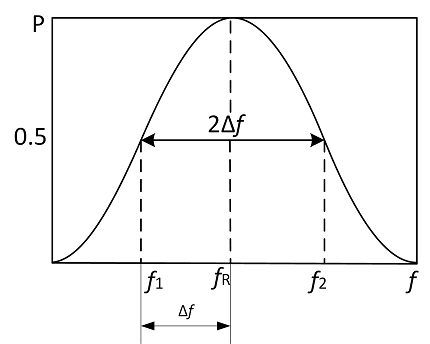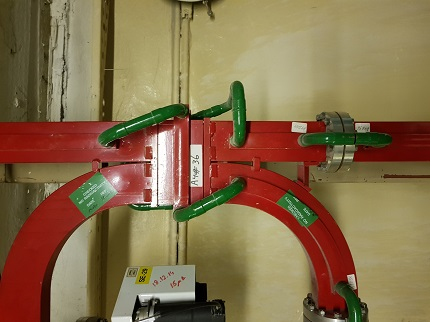﻿ Lab Work on RF equipment Официальный сайт УНЦ ОИЯИ

### Lab Work on RF technology

RF technology is widely used in accelerator technology (particles acceleration, charged ions generation, particle bunching, etc.).

High frequency technology differs from classic electronics: there is no distinction between elements (capacitor, inductance, and so on) and transmission line. Elements influencing wave propagation are often irregularities of the transmission line itself: plates, diaphragms, dowels, couplers, etc.

The Lab Work is aimed at training (either from scratch or for skills upgrading) of the shift personnel and particle accelerator service/maintenance staff. The Lab Work is aimed at introduction to the main RF technology elements and demonstration of the wave behavior in different conditions: without obstacles, with various hindrances (irregularities), and under the influence of the devices intended for wave behavior control (directional coupler and phase-shifter). Additionally, the Lab Work provides insight into the basic RF systems parameters and gives skills of their calculation and measurement using real equipment.

Prerequisites:

• basics of mathematical analysis, linear algebra and analytic geometry
• basics of physics: metric prefixes, basics of electricity
• basics of computer knowledge: MS Windows, MS Office
• appropriate glasses/lenses if the student has poor eyesight: the training includes work with small elements

The practice is nominally divided into theoretical and practical parts. The theoretical one, despite the name, implies working with equipment, but does not give any real RF problem-solving skills — its main task is to demonstrate the basic principles and definitions. Practical works give real hands-on experience.

### List of Lab Works (T — theoretical, P — practical):

 0. (T) Introduction Result: general idea of the RF techology and its usage, and means of RF waves transmission.1. (P) Introduction to the equipment Result: skill of working with the equipment: SRS SG384 RF generator, Tektronix TDS 540D oscilloscope, B6-9 microvoltmeter, KEYSIGHT E5071C vector analyzer, M3 RF power meter.2. (T) Determining of the wave length in the waveguide Result: understanding of the wave behavior in the feeder lines. Skill of calculating the wave length in the free space and feeder lines theoretically, as well as of measuring the wave length using various measurement lines (coaxial, flat coaxial, waveguide).3. (T) Matching quality coefficients Result: general idea of the waveguide irregularities (open waveguide, matched load, capacitive and inductive diaphragms), general idea of the key RF parameters: reflection coefficient (Г), standing wave ratio (SWR), and travelling wave ratio (TWR), and methods of their determination.4. (T) Total resistances Result: general idea of the active, reactive, and total resistance and conductivity. Skill of calculating the total resistance of different irregularities theoretically, and determining it using Smith chart and using the vector analyzer.5. (P) Reactive dowel Result: general idea of the reactive dowel and capacitive/inductive types of its conductivity reactive component.Skill of matching the transmission line using the vector analyzer.6. (P) Cavity resonator Result: general idea of the quality factor — important parameter of the accelerating systems (it defines how well the system can accumulate energy, and, therefore, how well it can transfer this energy to accelerating particles). Skill of calculating the cavity quality factor.6*. (P) Cyclotron frequency tuning Result: skill of the “cold tuning” of the inductively coupled quarter-wave cavity RF system, skill of calculating the capacitive trimmer for compensation of the minor frequency shifts.7. (P) Directional coupler Result: general idea of the directional coupler and its key parameters (directivity and attenuation coefficients); skill of calculating these coefficients on the basis of the measured power in the coupled waveguide. Skill of calculating the power in the main waveguide on the basis of the measured coupled power and known coupler parameters (real practical task).8. (P) Phase-shifter Result: general idea of the phase-shifter, their types. Skill of calibrating the phase-shifter (real practical task).9. (P) Attenuator Result: general idea of the attenuator, their types. Skill of calibrating the attenuator by the method of the successive substitution (real practical task).Full course is designed for 3 weeks. However, lab works duration highly depends on student's level and basic skills. Depending on that the amount of the lab works in the course may vary. It is also possible to shorten the course by removing some lab works.